# 四、训练模型

• 直接使用封闭方程进行求根运算，得到模型在当前训练集上的最优参数（即在训练集上使损失函数达到最小值的模型参数）

• 使用迭代优化方法：梯度下降（GD），在训练集上，它可以逐渐调整模型参数以获得最小的损失函数，最终，参数会收敛到和第一种方法相同的的值。同时，我们也会介绍一些梯度下降的变体形式：批量梯度下降（Batch GD）、小批量梯度下降（Mini-batch GD）、随机梯度下降（Stochastic GD），在第二部分的神经网络部分，我们会多次使用它们。

## 线性回归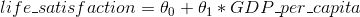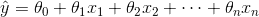• y_hat表示预测结果
• n表示特征的个数
• x[i]表示第i个特征的值
• θ[j]表示第j个参数（包括偏置项θ和特征权重值θ, θ, ..., θ[nj]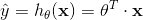• θ表示模型的参数向量包括偏置项θ和特征权重值θθ[n]
• θ^T表示向量θ的转置（行向量变为了列向量）
• x为每个样本中特征值的向量形式，包括xx[n]，而且x恒为 1
• θ^T · x表示θ^Tx的点积
• h[θ]表示参数为θ的假设函数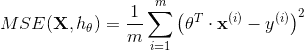### 正规方程(The Normal Equation)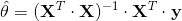• θ_hat指最小化损失θ的值
• y是一个向量，其包含了y^(1)y^(m)的值

import numpy as np
X = 2 * np.random.rand(100, 1)
y = 4 + 3 * X + np.random.randn(100, 1)X_b = np.c_[np.ones((100, 1)), X]
theta_best = np.linalg.inv(X_b.T.dot(X_b)).dot(X_b.T).dot(y)


>>> theta_best
array([[4.21509616],[2.77011339]])


>>> X_new = np.array([,])
>>> X_new_b = np.c_[np.ones((2, 1)), X_new]
>>> y_predict = X_new_b.dot(theta_best)
>>> y_predict
array([[4.21509616],[9.75532293]])


plt.plot(X_new,y_predict,"r-")
plt.plot(X,y,"b.")
plt.axis([0,2,0,15])
plt.show()>>> from sklearn.linear_model import LinearRegression
>>> lin_reg = LinearRegression()
>>> lin_reg.fit(X,y)
>>> lin_reg.intercept_, lin_reg.coef_
(array([4.21509616]),array([2.77011339]))
>>> lin_reg.predict(X_new)
array([[4.21509616],[9.75532293]])


## 梯度下降### 批量梯度下降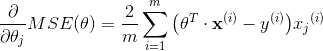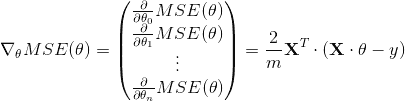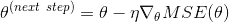eta = 0.1 # 学习率
n_iterations = 1000
m = 100

theta = np.random.randn(2,1) # 随机初始值

for iteration in range(n_iterations):
gradients = 2/m * X_b.T.dot(X_b.dot(theta) - y)
theta = theta - eta * gradients


>>> theta
array([[4.21509616],[2.77011339]])### 随机梯度下降n_epochs = 50
t0, t1 = 5, 50  #learning_schedule 的超参数

def learning_schedule(t):
return t0 / (t + t1)

theta = np.random.randn(2,1)

for epoch in range(n_epochs):
for i in range(m):
random_index = np.random.randint(m)
xi = X_b[random_index:random_index+1]
yi = y[random_index:random_index+1]
eta = learning_schedule(epoch * m + i)
theta = theta - eta * gradients


>>> theta
array([[4.21076011],[2.748560791]])from sklearn.linear_model import SGDRegressor
sgd_reg = SGDRegressor(n_iter=50, penalty=None, eta0=0.1)
sgd_reg.fit(X,y.ravel())


>>> sgd_reg.intercept_, sgd_reg.coef_
(array([4.18380366]),array([2.74205299]))


### 小批量梯度下降## 多项式回归

m = 100
X = 6 * np.random.rand(m, 1) - 3
y = 0.5 * X**2 + X + 2 + np.random.randn(m, 1)>>> from sklearn.preprocessing import PolynomialFeatures
>>> poly_features = PolynomialFeatures(degree=2,include_bias=False)
>>> X_poly = poly_features.fit_transform(X)
>>> X
array([-0.75275929])
>>> X_poly
array([-0.75275929, 0.56664654])


X_poly现在包含原始特征X并加上了这个特征的平方X^2。现在你可以在这个扩展训练集上使用LinearRegression模型进行拟合，如图 4-13：

>>> lin_reg = LinearRegression()
>>> lin_reg.fit(X_poly, y)
>>> lin_reg.intercept_, lin_reg.coef_
(array([ 1.78134581]), array([[ 0.93366893, 0.56456263]]))PolynomialFeatures(degree=d)把一个包含n个特征的数组转换为一个包含(n+d)!/(d!n!)特征的数组，n!表示n的阶乘，等于1 * 2 * 3 ... * n。小心大量特征的组合爆炸！

## 学习曲线from sklearn.metrics import mean_squared_error
from sklearn.model_selection import train_test_split

def plot_learning_curves(model, X, y):
X_train, X_val, y_train, y_val = train_test_split(X, y, test_size=0.2)
train_errors, val_errors = [], []
for m in range(1, len(X_train)):
model.fit(X_train[:m], y_train[:m])
y_train_predict = model.predict(X_train[:m])
y_val_predict = model.predict(X_val)
train_errors.append(mean_squared_error(y_train_predict, y_train[:m]))
val_errors.append(mean_squared_error(y_val_predict, y_val))
plt.plot(np.sqrt(train_errors), "r-+", linewidth=2, label="train")
plt.plot(np.sqrt(val_errors), "b-", linewidth=3, label="val")


lin_reg = LinearRegression()
plot_learning_curves(lin_reg, X, y)from sklearn.pipeline import Pipeline

polynomial_regression = Pipeline((
("poly_features", PolynomialFeatures(degree=10, include_bias=False)),
("sgd_reg", LinearRegression()),
))

plot_learning_curves(polynomial_regression, X, y)


• 在训练集上，误差要比线性回归模型低的多。
• 图中的两条曲线之间有间隔，这意味模型在训练集上的表现要比验证集上好的多，这也是模型过拟合的显著特点。当然，如果你使用了更大的训练数据，这两条曲线最后会非常的接近。• 偏差：泛化误差的这部分误差是由于错误的假设决定的。例如实际是一个二次模型，你却假设了一个线性模型。一个高偏差的模型最容易出现欠拟合。
• 方差：这部分误差是由于模型对训练数据的微小变化较为敏感，一个多自由度的模型更容易有高的方差（例如一个高阶多项式模型），因此会导致模型过拟合。
• 不可约误差：这部分误差是由于数据本身的噪声决定的。降低这部分误差的唯一方法就是进行数据清洗（例如：修复数据源，修复坏的传感器，识别和剔除异常值）。

## 线性模型的正则化

### 岭（Ridge）回归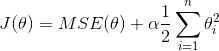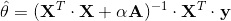>>> from sklearn.linear_model import Ridge
>>> ridge_reg = Ridge(alpha=1, solver="cholesky")
>>> ridge_reg.fit(X, y)
>>> ridge_reg.predict([[1.5]])
array([[ 1.55071465]]


>>> sgd_reg = SGDRegressor(penalty="l2")
>>> sgd_reg.fit(X, y.ravel())
>>> sgd_reg.predict([[1.5]])
array([[ 1.13500145]])


penalty参数指的是正则项的惩罚类型。指定l2表明你要在损失函数上添加一项：权重向量l2范数平方的一半，这就是简单的岭回归。

### Lasso 回归

Lasso 回归（也称 Least Absolute Shrinkage，或者 Selection Operator Regression）是另一种正则化版的线性回归：就像岭回归那样，它也在损失函数上添加了一个正则化项，但是它使用权重向量的l1范数而不是权重向量l2范数平方的一半。（如公式 4-10）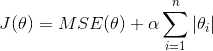Lasso 回归的一个重要特征是它倾向于完全消除最不重要的特征的权重（即将它们设置为零）。例如，右图中的虚线所示（α = 10^(-7)），曲线看起来像一条二次曲线，而且几乎是线性的，这是因为所有的高阶多项特征都被设置为零。换句话说，Lasso 回归自动的进行特征选择同时输出一个稀疏模型（即，具有很少的非零权重）。Lasso 损失函数在theta[i] = 0, i = 1, 2, ..., n处无法进行微分运算，但是梯度下降如果你使用子梯度向量g后它可以在任何θ[i] = 0的情况下进行计算。公式 4-11 是在 Lasso 损失函数上进行梯度下降的子梯度向量公式。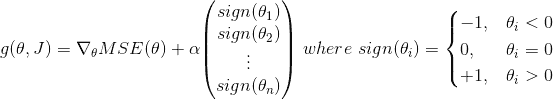>>> from sklearn.linear_model import Lasso
>>> lasso_reg = Lasso(alpha=0.1)
>>> lasso_reg.fit(X, y)
>>> lasso_reg.predict([[1.5]])
array([ 1.53788174]


### 弹性网络（ElasticNet）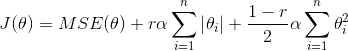>>> from sklearn.linear_model import ElasticNet
>>> elastic_net = ElasticNet(alpha=0.1, l1_ratio=0.5)
>>> elastic_net.fit(X, y)
>>> elastic_net.predict([[1.5]])
array([ 1.54333232])


### 早期停止法（Early Stopping）from sklearn.base import clone
sgd_reg = SGDRegressor(n_iter=1, warm_start=True, penalty=None,learning_rate="constant", eta0=0.0005)

minimum_val_error = float("inf")
best_epoch = None
best_model = None
for epoch in range(1000):
sgd_reg.fit(X_train_poly_scaled, y_train)
y_val_predict = sgd_reg.predict(X_val_poly_scaled)
val_error = mean_squared_error(y_val_predict, y_val)
if val_error < minimum_val_error:
minimum_val_error = val_error
best_epoch = epoch
best_model = clone(sgd_reg)


## 逻辑回归

### 概率估计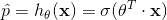Logistic 函数（也称为 logit），用σ()表示，其是一个 sigmoid 函数（图像呈 S 型），它的输出是一个介于 0 和 1 之间的数字。其定义如公式 4-14 和图 4-21 所示。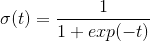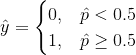### 训练和损失函数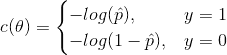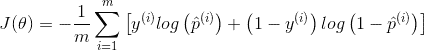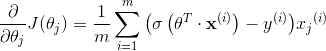### 决策边界>>> from sklearn import datasets
>>> list(iris.keys())
['data', 'target_names', 'feature_names', 'target', 'DESCR']
>>> X = iris["data"][:, 3:] # petal width
>>> y = (iris["target"] == 2).astype(np.int)


from sklearn.linear_model import LogisticRegression

log_reg = LogisticRegression()
log_reg.fit(X, y)


X_new = np.linspace(0, 3, 1000).reshape(-1, 1)
y_proba = log_reg.predict_proba(X_new)
plt.plot(X_new, y_proba[:, 1], "g-", label="Iris-Virginica")
plt.plot(X_new, y_proba[:, 0], "b--", label="Not Iris-Virginica")Virginica 花的花瓣宽度（用三角形表示）在 1.4 厘米到 2.5 厘米之间，而其他种类的花（由正方形表示）通常具有较小的花瓣宽度，范围从 0.1 厘米到 1.8 厘米。注意，它们之间会有一些重叠。在大约 2 厘米以上时，分类器非常肯定这朵花是 Virginica 花（分类器此时输出一个非常高的概率值），而在 1 厘米以下时，它非常肯定这朵花不是 Virginica 花（不是 Virginica 花有非常高的概率）。在这两个极端之间，分类器是不确定的。但是，如果你使用它进行预测（使用predict()方法而不是predict_proba()方法），它将返回一个最可能的结果。因此，在 1.6 厘米左右存在一个决策边界，这时两类情况出现的概率都等于 50%：如果花瓣宽度大于 1.6 厘米，则分类器将预测该花是 Virginica，否则预测它不是（即使它有可能错了）：

>>> log_reg.predict([[1.7], [1.5]])
array([1, 0])### Softmax 回归

Logistic 回归模型可以直接推广到支持多类别分类，不必组合和训练多个二分类器（如第 3 章所述）， 其称为 Softmax 回归或多类别 Logistic 回归。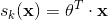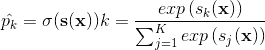• K表示有多少类
• s(x)表示包含样本x每一类得分的向量
• σ(s(x)[k])表示给定每一类分数之后，实例x属于第k类的概率• argmax运算返回一个函数取到最大值的变量值。 在这个等式，它返回使σ(s(x)[k])最大时的k的值

Softmax 回归分类器一次只能预测一个类（即它是多类的，但不是多输出的），因此它只能用于判断互斥的类别，如不同类型的植物。 你不能用它来识别一张照片中的多个人。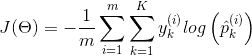• 如果对于第i个实例的目标类是k，那么y[k]^(i) = 1，反之y[k]^(i) = 0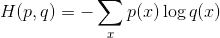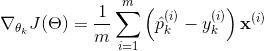X = iris["data"][:, (2, 3)] # petal length, petal width
y = iris["target"]

softmax_reg = LogisticRegression(multi_class="multinomial",solver="lbfgs", C=10)
softmax_reg.fit(X, y)


>>> softmax_reg.predict([[5, 2]])
array()
>>> softmax_reg.predict_proba([[5, 2]])
array([[ 6.33134078e-07, 5.75276067e-02, 9.42471760e-01]])是## 练习

1. 如果你有一个数百万特征的训练集，你应该选择哪种线性回归训练算法？
2. 假设你训练集中特征的数值尺度（scale）有着非常大的差异，哪种算法会受到影响？有多大的影响？对于这些影响你可以做什么？
3. 训练 Logistic 回归模型时，梯度下降是否会陷入局部最低点？
4. 在有足够的训练时间下，是否所有的梯度下降都会得到相同的模型参数？
5. 假设你使用批量梯度下降法，画出每一代的验证误差。当你发现验证误差一直增大，接下来会发生什么？你怎么解决这个问题？
6. 当验证误差升高时，立即停止小批量梯度下降是否是一个好主意？
7. 哪个梯度下降算法（在我们讨论的那些算法中）可以最快到达解的附近？哪个的确实会收敛？怎么使其他算法也收敛？
8. 假设你使用多项式回归，画出学习曲线，在图上发现学习误差和验证误差之间有着很大的间隙。这表示发生了什么？有哪三种方法可以解决这个问题？
9. 假设你使用岭回归，并发现训练误差和验证误差都很高，并且几乎相等。你的模型表现是高偏差还是高方差？这时你应该增大正则化参数α，还是降低它？
10. 你为什么要这样做：
11. 使用岭回归代替线性回归？
12. Lasso 回归代替岭回归？
13. 弹性网络代替 Lasso 回归？
14. 假设你想判断一副图片是室内还是室外，白天还是晚上。你应该选择二个逻辑回归分类器，还是一个 Softmax 分类器？
15. 在 Softmax 回归上应用批量梯度下降的早期停止法（不使用 Scikit-Learn）。